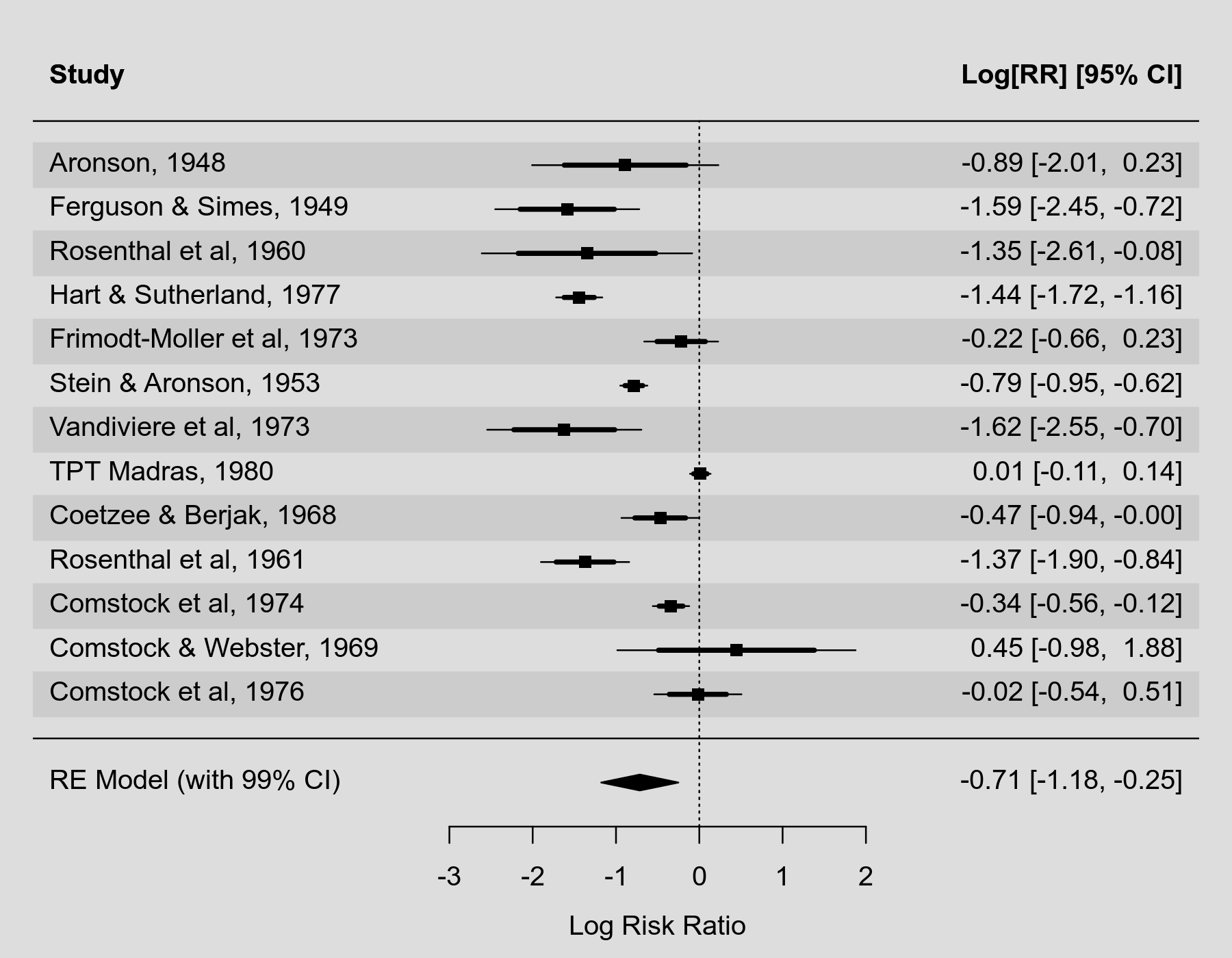#The metafor Package

A Meta-Analysis Package for R

### Site Tools

plots:forest_plot_with_multiple_cis

## Forest Plot with Multiple CIs

### Description

The question has come up how one can draw a forest plot where multiple confidence intervals with different confidence levels are shown for the same set of studies. To accomplish this, we can use a trick where we draw the same forest plot twice on top of each other (using par(new=TRUE)) and then adjust the line width (using lwd) so that the wider confidence intervals are drawn using a thicker line. The second time the plot is drawn, we suppress several elements (i.e., the points, annotations, the vertical reference line, the study labels, the x-axis and its label, and the horizontal line) so that these elements are not drawn twice.

A related question asked about the possibility to draw the confidence interval of the pooled estimate (from a random-effects model) using a different level than the one that is used for the studies. This can be accomplished by adding the pooled estimate to the plot using addpoly() and changing the level.

The example below illustrates both of these possibilities.

### Plot### Code

library(metafor)

### copy BCG vaccine meta-analysis data into 'dat'
dat <- dat.bcg

### calculate log risk ratios and corresponding sampling variances
dat <- escalc(measure="RR", ai=tpos, bi=tneg, ci=cpos, di=cneg, data=dat,
slab=paste(author, year, sep=", "))

### draw forest plot with 95% CIs for the studies
with(dat, forest(yi, vi, xlim=c(-8,6), ylim=c(-1.5,nrow(dat)+3),

### superimpose forest plot with 80% CIs for the studies
par(new=TRUE)
with(dat, forest(yi, vi, xlim=c(-8,6), ylim=c(-1.5,nrow(dat)+3), level=80, lwd=3,
psize=NA, efac=NA, annotate=FALSE, refline=NA, slab=NA,
xaxt="n", xlab="", lty=c("solid","blank")))

### fit random-effects model
res <- rma(yi, vi, data=dat)

### add pooled estimate to the forest plot with a 99% CI
addpoly(res, row=-1, level=99, mlab="RE Model (with 99% CI)", annotate=TRUE)

### add a horizontal line between the studies and the pooled estimate
abline(h=0)